Integrated Rate Laws

Learning Objectives

By the end of this module, you will be able to:

• Explain the form and function of an integrated rate law
• Perform integrated rate law calculations for zero-, first-, and second-order reactions
• Define half-life and carry out related calculations
• Identify the order of a reaction from concentration/time data

The rate laws we have seen thus far relate the rate and the concentrations of reactants. We can also determine a second form of each rate law that relates the concentrations of reactants and time. These are called integrated rate laws. We can use an integrated rate law to determine the amount of reactant or product present after a period of time or to estimate the time required for a reaction to proceed to a certain extent. For example, an integrated rate law is used to determine the length of time a radioactive material must be stored for its radioactivity to decay to a safe level.

Using calculus, the differential rate law for a chemical reaction can be integrated with respect to time to give an equation that relates the amount of reactant or product present in a reaction mixture to the elapsed time of the reaction. This process can either be very straightforward or very complex, depending on the complexity of the differential rate law. For purposes of discussion, we will focus on the resulting integrated rate laws for first-, second-, and zero-order reactions.

First-Order Reactions

An equation relating the rate constant k to the initial concentration [A]0 and the concentration [A]t present after any given time t can be derived for a first-order reaction and shown to be:

$\text{ln}\left(\frac{{\left[A\right]}_{t}}{{\left[A\right]}_{0}}\right)=-kt$

or

$\text{ln}\left(\frac{{\left[A\right]}_{0}}{{\left[A\right]}_{t}}\right)=kt$

Example 1: The Integrated Rate Law for a First-Order Reaction

The rate constant for the first-order decomposition of cyclobutane, C4H8 at 500 °C is 9.2 × 10−3 s−1:

${\text{C}}_{4}{\text{H}}_{8}\rightarrow{\text{2C}}_{2}{\text{H}}_{4}$

How long will it take for 80.0% of a sample of C4H8 to decompose?

Iodine-131 is a radioactive isotope that is used to diagnose and treat some forms of thyroid cancer. Iodine-131 decays to xenon-131 according to the equation:

$\text{I-131}\rightarrow\text{Xe-131}+\text{electron}$

The decay is first-order with a rate constant of 0.138 d−1. All radioactive decay is first order. How many days will it take for 90% of the iodine-131 in a 0.500 M solution of this substance to decay to Xe-131?

We can use integrated rate laws with experimental data that consist of time and concentration information to determine the order and rate constant of a reaction. The integrated rate law can be rearranged to a standard linear equation format:

$\begin{array}{ccc}\hfill \text{ln}\left[A\right]& =& \left(\text{-}k\right)\left(t\right)+\text{ln}{\left[A\right]}_{0}\hfill \\ \hfill y& =& mx+b\hfill \end{array}$

A plot of ln[A] versus t for a first-order reaction is a straight line with a slope of –k and an intercept of ln[A]0. If a set of rate data are plotted in this fashion but do not result in a straight line, the reaction is not first order in A.

Example 2: Determination of Reaction Order by Graphing

Show that the data in Figure 1 can be represented by a first-order rate law by graphing ln[H2O2] versus time. Determine the rate constant for the rate of decomposition of H2O2 from this data.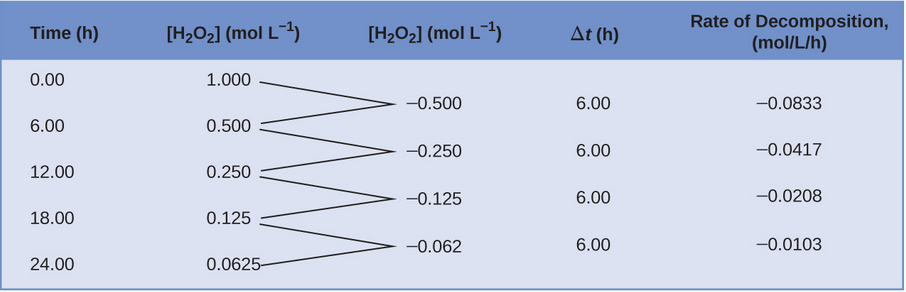Figure 1. The rate of decomposition of H2O2 in an aqueous solution decreases as the concentration of H2O2 decreases.

Graph the following data to determine whether the reaction $A\rightarrow B+C$ is first order.

Trial Time (s) [A]
1 4.0 0.220
2 8.0 0.144
3 12.0 0.110
4 16.0 0.088
5 20.0 0.074

Second-Order Reactions

The equations that relate the concentrations of reactants and the rate constant of second-order reactions are fairly complicated. We will limit ourselves to the simplest second-order reactions, namely, those with rates that are dependent upon just one reactant’s concentration and described by the differential rate law:

$\text{Rate}=k{\left[A\right]}^{2}$

For these second-order reactions, the integrated rate law is:

$\frac{1}{\left[A\right]}=kt+\frac{1}{{\left[A\right]}_{0}}$

where the terms in the equation have their usual meanings as defined above.

Example 3: The Integrated Rate Law for a Second-Order Reaction

The reaction of butadiene gas (C4H6) with itself produces C8H12 gas as follows:

${\text{2C}}_{4}{\text{H}}_{6}\text{(}g\text{)}\rightarrow{\text{C}}_{8}{\text{H}}_{12}\text{(}g\text{)}$

The reaction is second order with a rate constant equal to 5.76 × 10−2 L/mol/min under certain conditions. If the initial concentration of butadiene is 0.200 M, what is the concentration remaining after 10.0 min?

If the initial concentration of butadiene is 0.0200 M, what is the concentration remaining after 20.0 min?

The integrated rate law for our second-order reactions has the form of the equation of a straight line:

$\begin{array}{ccc}\hfill \frac{1}{\left[A\right]}& =& kt+\frac{1}{{\left[A\right]}_{0}}\hfill \\ \hfill y& =& mx+b\hfill \end{array}$

A plot of $\frac{1}{\left[A\right]}$ versus t for a second-order reaction is a straight line with a slope of k and an intercept of $\frac{1}{{\left[A\right]}_{0}}$. If the plot is not a straight line, then the reaction is not second order.

Example 4: Determination of Reaction Order by Graphing

Test the data given to show whether the dimerization of C4H6 is a first- or a second-order reaction.

Does the following data fit a second-order rate law?

Trial Time (s) [A] (M)
1 5 0.952
2 10 0.625
3 15 0.465
4 20 0.370
5 25 0.308
6 35 0.230

Zero-Order Reactions

For zero-order reactions, the differential rate law is:

$\text{Rate}=k{\left[A\right]}^{0}=k$

A zero-order reaction thus exhibits a constant reaction rate, regardless of the concentration of its reactants.

The integrated rate law for a zero-order reaction also has the form of the equation of a straight line:

$\begin{array}{ccc}\hfill \left[A\right]& =& {-}kt+{\left[A\right]}_{0}\hfill \\ \hfill y& =& mx+b\hfill \end{array}$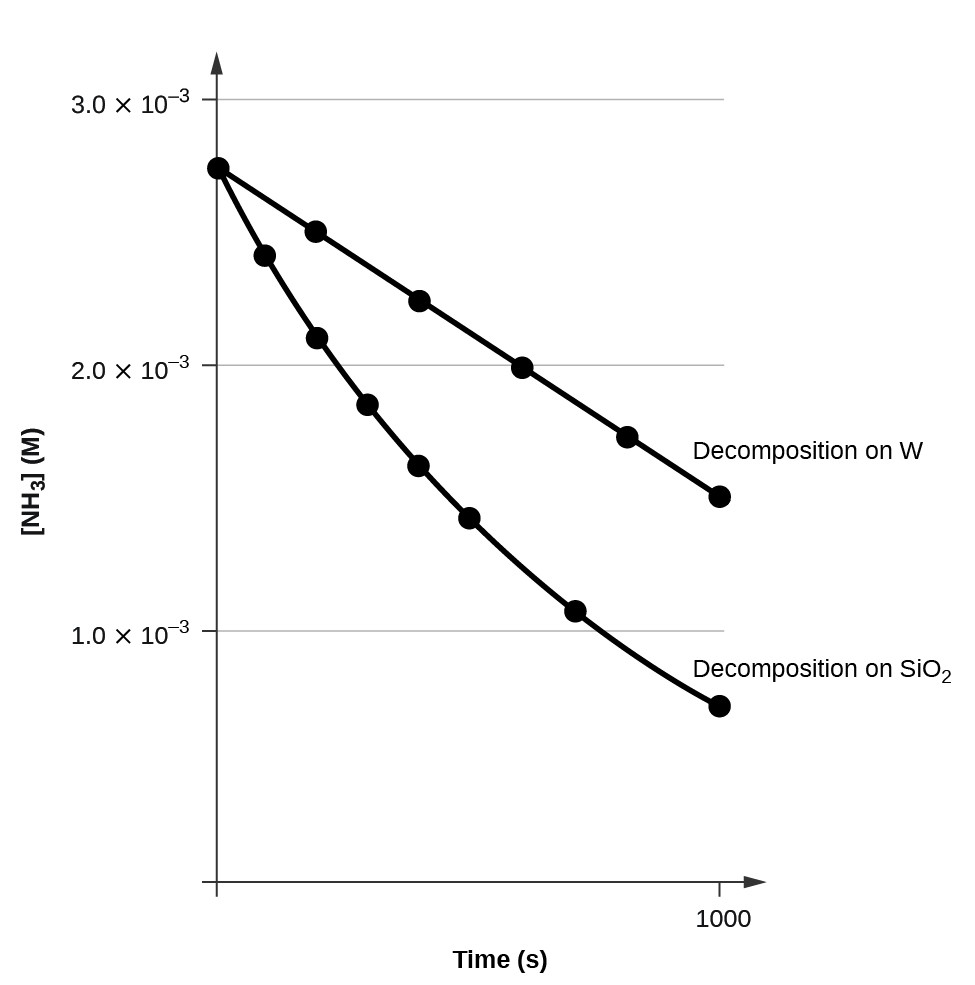Figure 4. The decomposition of NH3 on a tungsten (W) surface is a zero-order reaction, whereas on a quartz (SiO2) surface, the reaction is first order.

A plot of [A] versus t for a zero-order reaction is a straight line with a slope of −k and an intercept of [A]0. Figure 4 shows a plot of [NH3] versus t for the decomposition of ammonia on a hot tungsten wire and for the decomposition of ammonia on hot quartz (SiO2). The decomposition of NH3 on hot tungsten is zero order; the plot is a straight line. The decomposition of NH3 on hot quartz is not zero order (it is first order). From the slope of the line for the zero-order decomposition, we can determine the rate constant:

slope = −k = 1.3110−6 mol/L/s

The Half-Life of a Reaction

The half-life of a reaction (t1/2) is the time required for one-half of a given amount of reactant to be consumed. In each succeeding half-life, half of the remaining concentration of the reactant is consumed. Using the decomposition of hydrogen peroxide in Figure 1 as an example, we find that during the first half-life (from 0.00 hours to 6.00 hours), the concentration of H2O2 decreases from 1.000 M to 0.500 M. During the second half-life (from 6.00 hours to 12.00 hours), it decreases from 0.500 M to 0.250 M; during the third half-life, it decreases from 0.250 M to 0.125 M. The concentration of H2O2 decreases by half during each successive period of 6.00 hours. The decomposition of hydrogen peroxide is a first-order reaction, and, as can be shown, the half-life of a first-order reaction is independent of the concentration of the reactant. However, half-lives of reactions with other orders depend on the concentrations of the reactants.

First-Order Reactions

We can derive an equation for determining the half-life of a first-order reaction from the alternate form of the integrated rate law as follows:

$\begin{array}{lll}\hfill \mathrm{ln}\frac{{\left[A\right]}_{0}}{\left[A\right]}& =& kt\hfill \\ \hfill t& =& \mathrm{ln}\frac{{\left[A\right]}_{0}}{\left[A\right]}\times \frac{1}{k}\hfill \end{array}$

If we set the time t equal to the half-life, ${t}_{1\text{/}2},$ the corresponding concentration of A at this time is equal to one-half of its initial concentration. Hence, when $t={t}_{1\text{/}2},$ $\left[A\right]=\frac{1}{2}{\left[A\right]}_{0}$.

Therefore:

$\begin{array}{cc}\hfill {t}_{1\text{/}2}& =\mathrm{ln}\frac{{\left[A\right]}_{0}}{\frac{1}{2}{\left[A\right]}_{0}}\times \frac{1}{k}\hfill \\ & =\mathrm{ln}2\times \frac{1}{k}=0.693\times \frac{1}{k}\hfill \end{array}$

Thus:

${t}_{1\text{/}2}=\frac{0.693}{k}$

We can see that the half-life of a first-order reaction is inversely proportional to the rate constant k. A fast reaction (shorter half-life) will have a larger k; a slow reaction (longer half-life) will have a smaller k.

Example 5: Calculation of a First-order Rate Constant using Half-Life

Calculate the rate constant for the first-order decomposition of hydrogen peroxide in water at 40 °C, using the data given in Figure 5.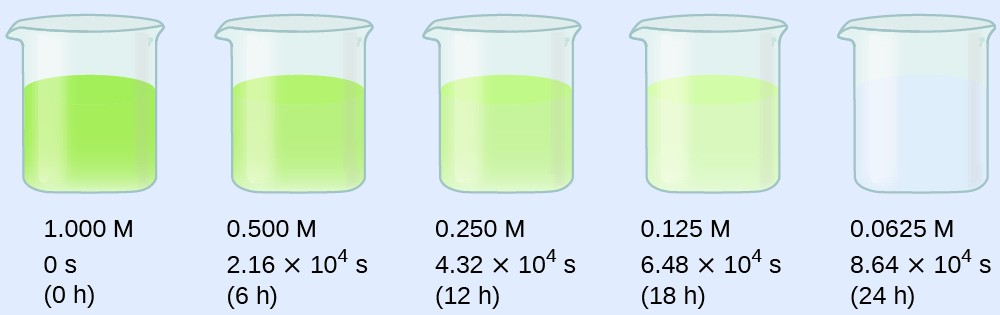Figure 5. The decomposition of H2O2 (2H2O2 → 2H2O + O2) at 40 °C is illustrated. The intensity of the color symbolizes the concentration of H2O2 at the indicated times; H2O2 is actually colorless.

The first-order radioactive decay of iodine-131 exhibits a rate constant of 0.138 d−1. What is the half-life for this decay?

Second-Order Reactions

We can derive the equation for calculating the half-life of a second order as follows:

$\frac{1}{\left[A\right]}=kt+\frac{1}{{\left[A\right]}_{0}}$

or

$\frac{1}{\left[A\right]}-\frac{1}{{\left[A\right]}_{0}}=kt$

If $t={t}_{1\text{/}2}$, then $\left[A\right]=\frac{1}{2}{\left[A\right]}_{0}$, and we can write:

$\begin{array}{ccc}\hfill \frac{1}{\frac{1}{2}{\left[A\right]}_{0}}-\frac{1}{{\left[A\right]}_{0}}& =& k{t}_{1\text{/}2}\hfill \\ \hfill 2{\left[A\right]}_{0}-\frac{1}{{\left[A\right]}_{0}}& =& k{t}_{1\text{/}2}\hfill \\ \hfill \frac{1}{{\left[A\right]}_{0}}& =& k{t}_{1\text{/}2}\hfill \end{array}$

Thus:

${t}_{1\text{/}2}=\frac{1}{k{\left[A\right]}_{0}}$

For a second-order reaction, ${t}_{1\text{/}2}$ is inversely proportional to the concentration of the reactant, and the half-life increases as the reaction proceeds because the concentration of reactant decreases. Consequently, we find the use of the half-life concept to be more complex for second-order reactions than for first-order reactions. Unlike with first-order reactions, the rate constant of a second-order reaction cannot be calculated directly from the half-life unless the initial concentration is known.

Zero-Order Reactions

We can derive an equation for calculating the half-life of a zero order reaction as follows:

$\left[A\right]={-}kt+{\left[A\right]}_{0}$

When half of the initial amount of reactant has been consumed $t={t}_{1\text{/}2}$ and $\left[A\right]=\frac{{\left[A\right]}_{0}}{2}.$ Thus:

$\begin{array}{ccc}\hfill \frac{{\left[\text{A}\right]}_{0}}{2}& =& {-}k{t}_{1\text{/}2}+{\left[\text{A}\right]}_{0}\hfill \\ \hfill k{t}_{1\text{/}2}& =& \frac{{\left[\text{A}\right]}_{0}}{2}\hfill \end{array}$

and

${t}_{1\text{/}2}=\text{}\frac{{\left[A\right]}_{0}}{2k}$

The half-life of a zero-order reaction increases as the initial concentration increases.

Equations for both differential and integrated rate laws and the corresponding half-lives for zero-, first-, and second-order reactions are summarized in Table 1.

Table 1. Summary of Rate Laws for Zero-, First-, and Second-Order Reactions
Zero-Order First-Order Second-Order
rate law rate = k rate = k[A] rate = k[A]2
units of rate constant M s−1 s−1 M−1 s−1
integrated rate law [A] = −kt + [A]0 ln [A] = −kt + ln[A]0 $\frac{1}{\left[A\right]}=kt+\left(\frac{1}{{\left[A\right]}_{0}}\right)$
plot needed for linear fit of rate data [A] vs. t ln [A] vs. t $\frac{1}{\left[A\right]}$ vs. t
relationship between slope of linear plot and rate constant k = −slope k = −slope k = +slope
half-life ${t}_{1\text{/}2}=\frac{{\left[A\right]}_{0}}{2k}$ ${t}_{1\text{/}2}=\frac{0.693}{k}$ ${t}_{1\text{/}2}=\frac{1}{{\left[A\right]}_{0}k}$

Key Concepts and Summary

Differential rate laws can be determined by the method of initial rates or other methods. We measure values for the initial rates of a reaction at different concentrations of the reactants. From these measurements, we determine the order of the reaction in each reactant. Integrated rate laws are determined by integration of the corresponding differential rate laws. Rate constants for those rate laws are determined from measurements of concentration at various times during a reaction.

The half-life of a reaction is the time required to decrease the amount of a given reactant by one-half. The half-life of a zero-order reaction decreases as the initial concentration of the reactant in the reaction decreases. The half-life of a first-order reaction is independent of concentration, and the half-life of a second-order reaction decreases as the concentration increases.

Key Equations

• integrated rate law for zero-order reactions: $\left[A\right]={-}kt+{\left[A\right]}_{0},$ ${t}_{1\text{/}2}=\frac{{\left[A\right]}_{0}}{2k}$
• integrated rate law for first-order reactions: $\mathrm{ln}\left[A\right]={-}kt+{\left[A\right]}_{0},\text{}{t}_{1\text{/}2}=\frac{0.693}{k}$
• integrated rate law for second-order reactions: $\frac{1}{\left[A\right]}=kt+\frac{1}{{\left[A\right]}_{0}},$ ${t}_{1\text{/}2}=\frac{1}{{\left[A\right]}_{0}k}$

Exercises

1. Describe how graphical methods can be used to determine the order of a reaction and its rate constant from a series of data that includes the concentration of A at varying times.
2. Use the data provided to graphically determine the order and rate constant of the following reaction: ${\text{SO}}_{2}{\text{Cl}}_{2}\rightarrow{\text{SO}}_{2}+{\text{Cl}}_{2}$
Time (s) [SO2Cl2] (M)
0 0.100
5.00 × 103 0.0896
1.00 × 104 0.0802
1.50 × 104 0.0719
2.50 × 104 0.0577
3.00 × 104 0.0517
4.00 × 104 0.0415
3. Use the data provided in a graphical method to determine the order and rate constant of the following reaction:$2P\rightarrow Q+W$
Time (s) [P] (M)
9.0 1.077 × 10-3
13.0 1.068 × 10-3
18.0 1.055 × 10-3
22.0 1.046 × 10-3
25.0 1.039 × 10-3
4. Pure ozone decomposes slowly to oxygen, ${\text{2O}}_{3}\left(g\right)\rightarrow{\text{3O}}_{2}\left(g\right).$ Use the data provided in a graphical method and determine the order and rate constant of the reaction.
Time (h) [O3] (M)
0 1.00 × 10−5
2.0 × 10−3 4.98 × 10−6
7.6 × 10−3 2.07 × 10−6
1.00 × 10−4 1.66 × 10−6
1.23 × 10−4 1.39 × 10−6
1.43 × 10−4 1.22 × 10−6
1.70 × 10−4 1.05 × 10−6
5. From the given data, use a graphical method to determine the order and rate constant of the following reaction:$2X\rightarrow Y+Z$
Time (s) [X] (M)
5.0 0.0990
10.0 0.0497
15.0 0.0332
20.0 0.0249
25.0 0.0200
30.0 0.0166
35.0 0.0143
40.0 0.0125
6. What is the half-life for the first-order decay of phosphorus-32 $\left({}_{15}^{32}\text{P}\rightarrow{}_{16}^{32}\text{S}+{\text{e}}^{-}\right)$ The rate constant for the decay is 4.85 × 10−2 day −1.
7. What is the half-life for the first-order decay of carbon-14? $\left({}_{14}^{6}\text{C}\rightarrow{}_{14}^{7}\text{N}+{\text{e}}^{-}\right)$ The rate constant for the decay is 1.21 × 10−4 year−1.
8. What is the half-life for the decomposition of NOCl when the concentration of NOCl is 0.15 M? The rate constant for this second-order reaction is 8.0 × 10-8 L/mol/s.
9. What is the half-life for the decomposition of O3 when the concentration of O3 is 2.35 × 10−6M? The rate constant for this second-order reaction is 50.4 L/mol/h.
10. The reaction of compound A to give compounds C and D was found to be second-order in A. The rate constant for the reaction was determined to be 2.42 L/mol/s. If the initial concentration is 0.500 mol/L, what is the value of t1/2?
11. The half-life of a reaction of compound A to give compounds D and E is 8.50 min when the initial concentration of A is 0.150 mol/L. How long will it take for the concentration to drop to 0.0300 mol/L if the reaction is (a) first order with respect to A or (b) second order with respect to A?
12. Some bacteria are resistant to the antibiotic penicillin because they produce penicillinase, an enzyme with a molecular weight of 3 × 104 g/mol that converts penicillin into inactive molecules. Although the kinetics of enzyme-catalyzed reactions can be complex, at low concentrations this reaction can be described by a rate equation that is first order in the catalyst (penicillinase) and that also involves the concentration of penicillin. From the following data: 1.0 L of a solution containing 0.15 µg (0.15 × 10−6 g) of penicillinase, determine the order of the reaction with respect to penicillin and the value of the rate constant.
[Penicillin] (M) Rate (mol/L/min)
2.0 × 10−6 1.0 × 10−10
3.0 × 10−6 1.5 × 10−10
4.0 × 10−6 2.0 × 10−10
13. Both technetium-99 and thallium-201 are used to image heart muscle in patients with suspected heart problems. The half-lives are 6 h and 73 h, respectively. What percent of the radioactivity would remain for each of the isotopes after 2 days (48 h)?
14. There are two molecules with the formula C3H6. Propene, CH3CH=CH2, is the monomer of the polymer polypropylene, which is used for indoor-outdoor carpets. Cyclopropane is used as an anesthetic: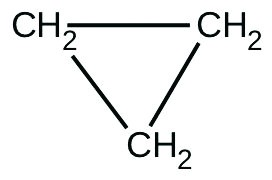When heated to 499 °C, cyclopropane rearranges (isomerizes) and forms propene with a rate constant of $5.95\times {10}^{-4}{s}^{-1}$. What is the half-life of this reaction? What fraction of the cyclopropane remains after 0.75 h at 499.5 °C?
15. Fluorine-18 is a radioactive isotope that decays by positron emission to form oxygen-18 with a half-life of 109.7 min. (A positron is a particle with the mass of an electron and a single unit of positive charge; the equation is ${}_{9}^{18}\text{F}\rightarrow{}_{8}^{18}\text{O}+{\text{e}}^{+}.$ ) Physicians use 18F to study the brain by injecting a quantity of fluoro-substituted glucose into the blood of a patient. The glucose accumulates in the regions where the brain is active and needs nourishment.
1. What is the rate constant for the decomposition of fluorine-18?
2. If a sample of glucose containing radioactive fluorine-18 is injected into the blood, what percent of the radioactivity will remain after 5.59 h?
3. How long does it take for 99.99% of the 18F to decay?
16. Suppose that the half-life of steroids taken by an athlete is 42 days. Assuming that the steroids biodegrade by a first-order process, how long would it take for $\frac{1}{64}$ of the initial dose to remain in the athlete’s body?
17. Recently, the skeleton of King Richard III was found under a parking lot in England. If tissue samples from the skeleton contain about 93.79% of the carbon-14 expected in living tissue, what year did King Richard III die? The half-life for carbon-14 is 5730 years.
18. Nitroglycerine is an extremely sensitive explosive. In a series of carefully controlled experiments, samples of the explosive were heated to 160 °C and their first-order decomposition studied. Determine the average rate constants for each experiment using the following data:
Initial [C3H5N3O9] (M) t (s) % Decomposed
4.88 300 52.0
3.52 300 52.9
2.29 300 53.2
1.81 300 53.9
5.33 180 34.6
4.05 180 35.9
2.95 180 36.0
1.72 180 35.4
19. For the past 10 years, the unsaturated hydrocarbon 1,3-butadiene (CH2=CH–CH=CH2) has ranked 38th among the top 50 industrial chemicals. It is used primarily for the manufacture of synthetic rubber. An isomer exists also as cyclobutene: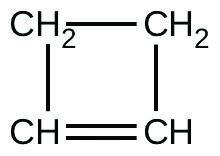The isomerization of cyclobutene to butadiene is first-order and the rate constant has been measured as 2.0 × 10−4 s−1 at 150°C in a 0.53-L flask. Determine the partial pressure of cyclobutene and its concentration after 30.0 minutes if an isomerization reaction is carried out at 150°C with an initial pressure of 55 torr.

Glossary

half-life of a reaction (tl/2): time required for half of a given amount of reactant to be consumed

integrated rate law: equation that relates the concentration of a reactant to elapsed time of reaction